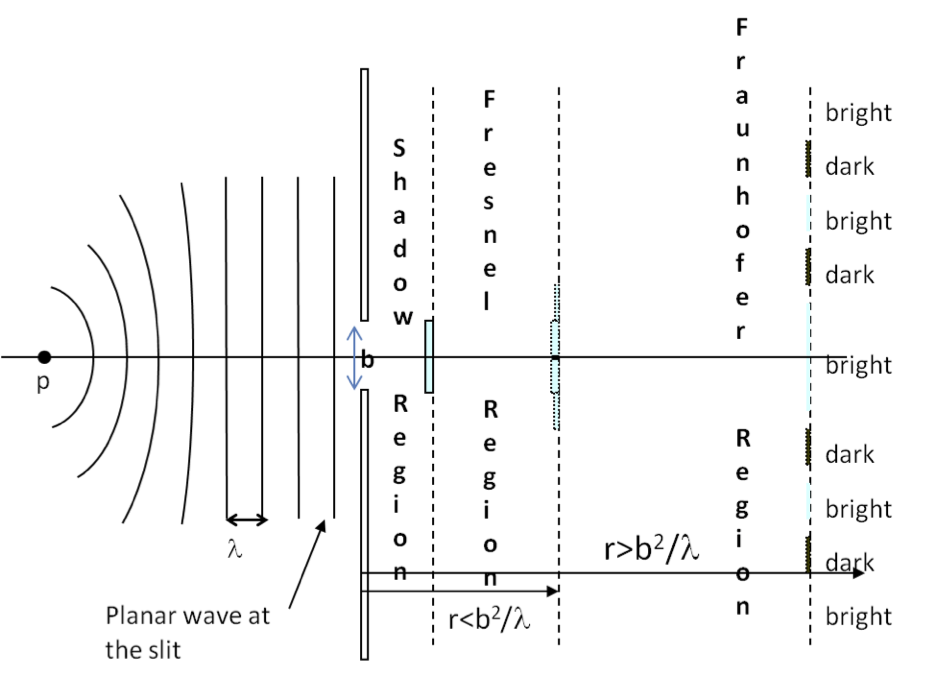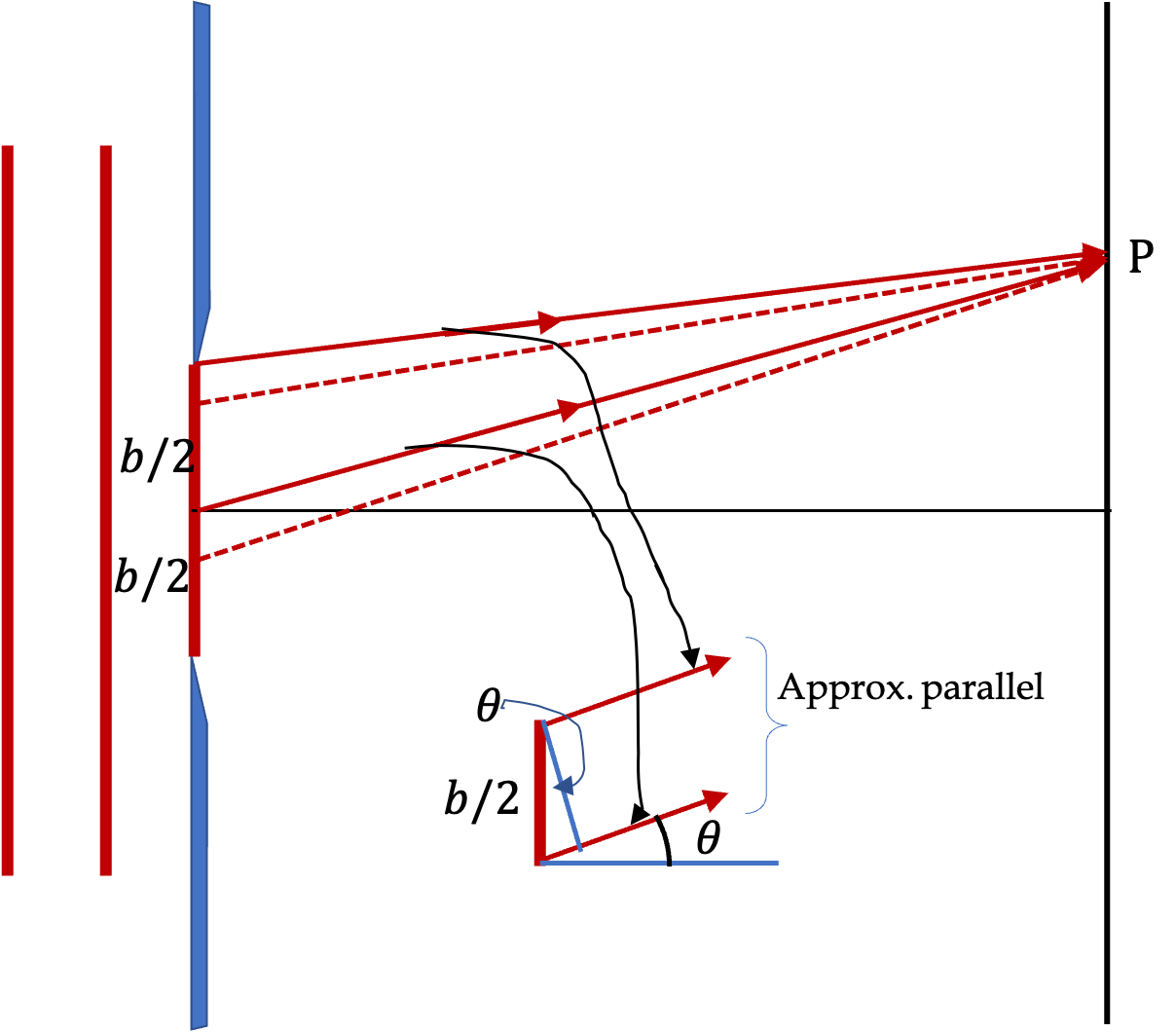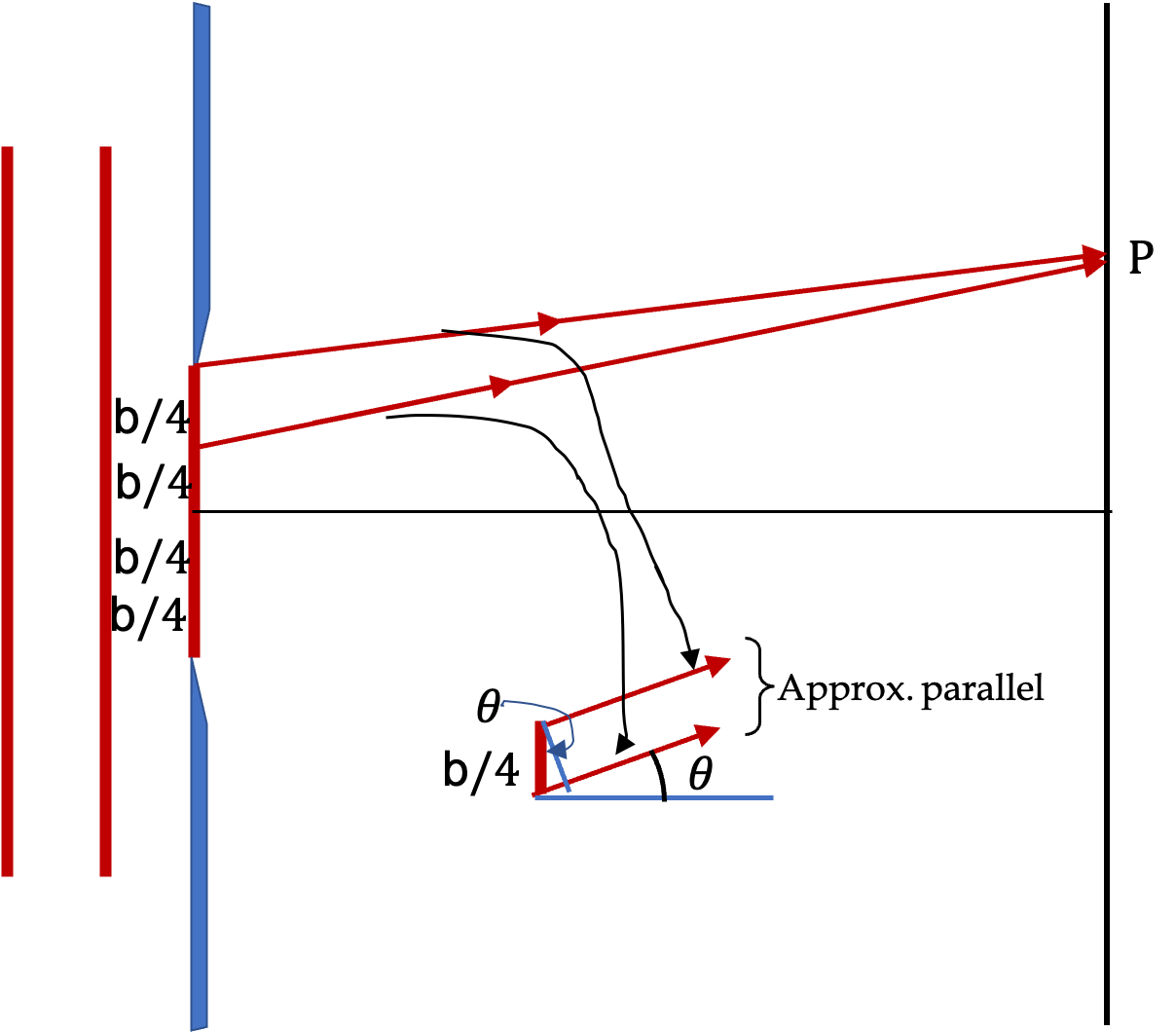## Section51.3Diffraction Through a Single Slit

In this section we study the diffraction phenomenon in more detail. As our first example, we consider a light source in front of a narrow slit in an opaque material as shown in Figure 51.3.1. Suppose the light source is either far away or at the focal plane of a lens so that the waves are planar at the slit.Figure 51.3.1. Shadow, Fresnel and Fraunhofer diffraction regions and patterns from a horizontal slit in front of a point source placed far away from the slit of width $b\text{.}$

We place a screen behind the slit to observe the diffraction pattern there when it is placed at different distances from the slit. If the screen is placed immediately behind the slit, we find a shadow of the slit on the screen. When we move the screen further out, the shadow develops fringes whose pattern changes as you move the screen further out. These patterns are called Fresnel or near-field diffraction}. Moving the screen further away from the slit you reach a region where the patterns stabilize and although they spread out more as you move the screen further away, the pattern remains the same. We call this pattern the Fraunhofer or far-field diffraction after Joseph von Fraunhofer, who built the first diffraction grating consisting of 260 closely, spaced parallel wires in 1821, and measured wavelengths of colored lights.

In the following, we will study a trick that lets us predict the directions for diffraction minima. In the next section we will study variation of intensity in a diffraction pattern. We can use the formula of intensity to find the direction of diffraction maxima as well as minima and the width of the peaks corresponding to the maxima.

### Subsection51.3.1Minima in a Single-slit Diffraction

How can we predict the locations of bright and dark circles on the screen of a single-slit Fraunhofer diffraction? It turns out that we can find the minima of the diffraction on the screen by a clever procedure rather than looking at how intensity of the wave varies with the direction. The trick is to imagine the wavefront at the slit as consisting of infinite number of wave sources and try to pair up two points whose waves will produce a destructive interference on the screen.

For instance, say we divide up the slit into two equal zones, we obtain one set of pairs of sources which consist of a point at the top of the upper zone and a point at the top of the lower zone, the next point of the top zone is paired with the corresponding point of the lower zone, etc., as illustrated in Figure 51.3.2.Figure 51.3.2. The first-order minimum - the path difference must equal half-wavelength for the paired waves to be out-of phase.

Since, the screen is far away, all the rays will be approximately parallel there and the path differences between the members of every pair will be same, regardless of their location in the zones. Thus if the path difference of one of these pairs is equal to half a wavelength, they will all interfere destructively at the screen.

We can work out the path difference from the triangle in Figure 51.3.2. With the slit width $b\text{,}$ the path difference between rays in the direction $\theta$ from the top of one zone and and the top of the zone below it will be

\begin{equation*} \frac{b}{2}\, \sin\theta. \end{equation*}

To be destructive, this will have to equal to $\lambda_2\text{.}$ You might say that we can capure this by making this equal to an odd multiple of $\lambda/2\text{,}$ but this trick does not work that way. To find the other diffraction minima, the trick is to divide th wavefront at the slit into 4 parts, 8 parts, 16 parts, etc., and make sure that the path difference between top of one zone and the top of the immediate next zone is $\pm \lambda/2\text{.}$

\begin{equation*} \frac{b}{2}\, \sin\theta = \pm \frac{\lambda}{2}. \end{equation*}

Simplifying this formula we get, as we will recognize as $m^\prime=\pm 1$ diffraction minimum when we study the intensity below,

\begin{equation} b\sin\theta = \pm \lambda.\tag{51.3.1} \end{equation}

Similarly, if you divide up the wave at the slit into four equal zones as shown in Figure 51.3.3.Figure 51.3.3. The second-order minimum - the path difference must equal half-wavelength for the paired waves to be out-of phase.

Let us label the zones 1, 2, 3 and 4,. We ensure that (1,2) cancel each other and (3,4) cancel each other. Since, the screen is supposed to be far away, we will get the same condition for the two pairs.

\begin{equation*} \frac{b}{4}\, \sin\theta = \pm \frac{\lambda}{2}. \end{equation*}

Simplifying this formula we get, as we will recognize as $m^\prime=\pm 2$ diffraction minimum when we study the intensity below,

\begin{equation} b\sin\theta = \pm2\lambda.\tag{51.3.2} \end{equation}

You can generalize your results to

\begin{equation*} b\sin\theta = m^\prime \lambda, \ \ \ (m^\prime = \pm 1, \pm 2, \pm 3, \cdots.) \end{equation*}

Light of wavelength $550\text{ nm}$ passes through a slit of width $2.0\:\mu\text{m}\text{.}$ Find the location of $m^\prime=-2,\ -1,\ 1,\ 2$ minima on a screen $50\text{ cm}$ away in terms of (a) angles subtendend with the horizontal direction from the center of the slit, and (b) $y$ coordinates of the positions of the dark bands using a $y$ axis pointed up with origin at the center of the central maximum.

Hint

(a Use the condition for minima. (b) Use $y=L\tan\theta\text{.}$

(a) $16^{\circ},\ 33.4^{\circ}\text{,}$ (b) $\pm 14\ \textrm{cm},\ \pm 33\ \textrm{cm}$ .

Solution 1 (a)

(a) The minima are given by the following condition

\begin{equation*} b\sin\theta = m^\prime \lambda \ \ (m^\prime = \pm 1, \pm 2, \pm 3, \cdots)\ \ \text{(destructive)}. \end{equation*}

Hence, the four minima around the central maxima will have $m^\prime = \pm 1, \pm 2\text{.}$ The corresponding directions from the slit are

\begin{align*} \amp \theta_{\pm 1} = \pm\sin\left( \frac{\lambda}{b}\right) = \pm 16^{\circ}\\ \amp \theta_{\pm 2} = \pm\sin\left( \frac{2\lambda}{b}\right) = \pm 33.4^{\circ} \end{align*}

Pictorially, the directions from the slit for the minima are illustrated in Figure 51.3.5.

The location of the diffraction minima on the screen can be deduced from the right-angled triangles. Let the $y$-axis be pointed up on the screen, then we will have
Denote the positions of the four minima by $y_{+1}\text{,}$ $y_{-1}\text{,}$ $y_{+2}$ and $y_{-2}\text{.}$